DAX time intelligence functions such as year-to-date (YTD), year-over-year (YOY), and several others come in handy when writing certain measures. Defining a date table containing all the dates in one year in order to use DAX time intelligence functions is considered a best practice. However, the presence of “future” dates in the date table can have the side effect of having measures that display values for those future dates – which might be confusing. Depending on the requirements, it might be necessary to limit the date range so that a measure does not show future dates and uses the same number of days in a comparison. The following sections describe different techniques to ensure correct results.

## Defining the issue with future dates

The first step is to show the issue when standard calculations display undesired values in future dates. Consider this measure for the year-to-date (YTD) calculation of Sales Amount:

```Sales YTD visible :=
CALCULATE (
[Sales Amount],
DATESYTD ( 'Date'[Date] )
)
```

In the sample data model used for this article, there are transactions between January 1, 2007 and August 7, 2009. Therefore, the last date to consider in the calculation should be August 7, 2009.

This is the resulting report: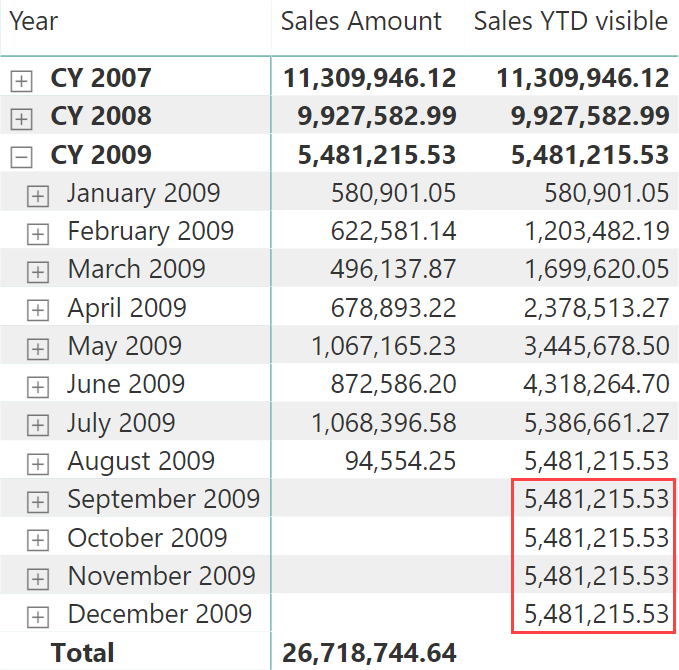The rows between September 2009 and December 2009 should not be visible. The goal here is to display a blank value in these out-of-range, “future” months.

A similar issue exists for the year-over-year calculation (YOY). Even though the measure tries to show a blank value in case of missing values in current or previous year, the amounts for August 2009 and for CY 2009 might be considered wrong.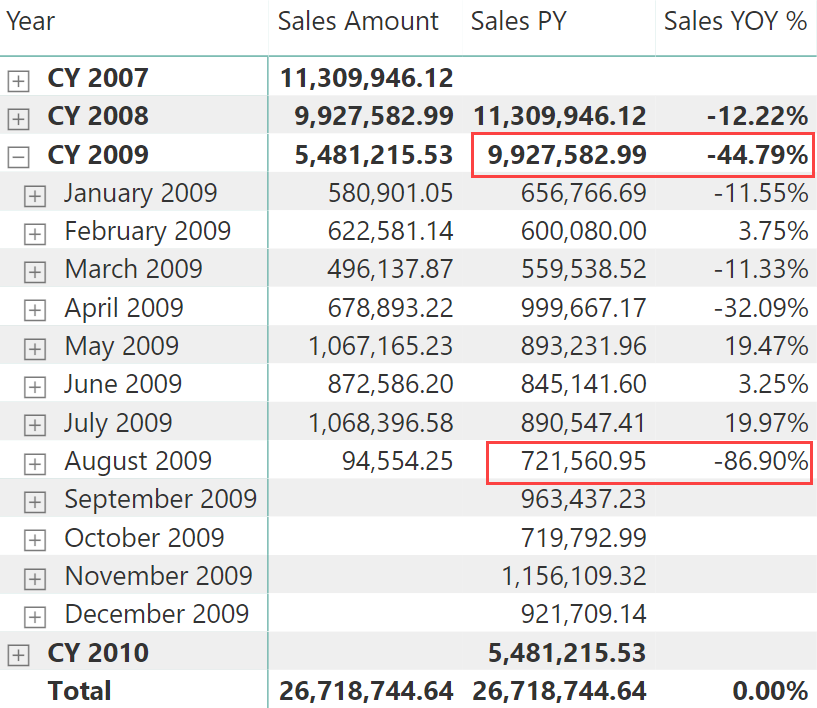The definition of Sales YOY % depends on Sales PY, as shown in the following code:

```Sales YOY % =
VAR CurrentSales = [Sales Amount]
VAR PreviousSales = [Sales PY]
VAR DeltaSales = CurrentSales - PreviousSales
VAR Result =
IF (
NOT ISBLANK ( CurrentSales ),
DIVIDE ( DeltaSales, PreviousSales )
)
RETURN Result

Sales PY =
CALCULATE (
[Sales Amount],
SAMEPERIODLASTYEAR ( 'Date'[Date] )
)
```

The Sales PY measure computes the values of Sales Amount for the same period in the previous year through the SAMEPERIODLASTYEAR function. However, the Sales PY measure returns the entire amount of August 2008, even though there are only 7 days with sales in August 2009. The following screenshot shows that the Sales PY amount for August 2009 includes all the days without sales in 2009 that have an amount in the corresponding day of 2008. This also impacts the CY 2009 calculation, which includes all the months until December.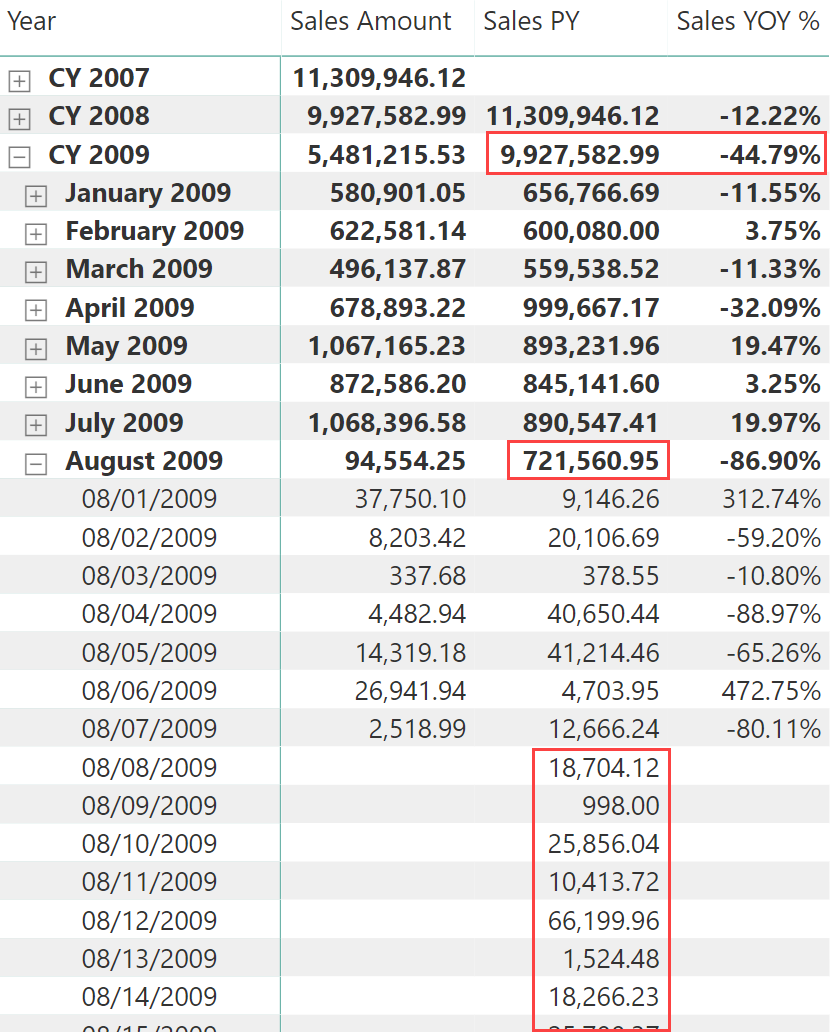The right value for Sales PY for August 2009 should be 128,866.59 instead of 721,560.95. Therefore, Sales YOY % displays an incorrect value because it uses Sales PY in its internal calculation.

The goal is to compute Sales PY with only the values found on the first 7 days of August 2008, and return blank for dates and months greater than August 7, 2009.

## Filtering dates through a calculated column

The simplest and most effective technique is to create a calculated column that marks the dates that are less than or equal to the last date that should be visible. For example, in a model with a Sales table containing transactions, the following calculated column can be created in the Date table:

```DatesWithSales =
'Date'[Date] <= MAX ( Sales[Order Date] )
```

The calculated column is automatically updated as soon as the Sales table is refreshed. All the dates that should not be visible in the reports have a FALSE value.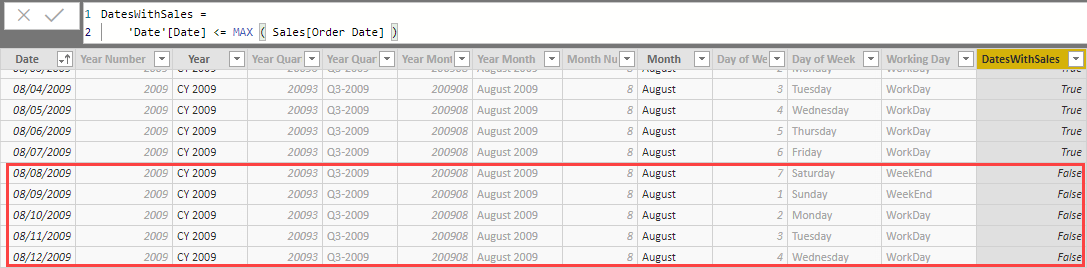When this column is available, all the time intelligence functions can behave as expected by just implementing a simple pattern. Instead of writing:

```CALCULATE (
<measure>,
<time_intelligence_function> ( 'Date'[Date] )
)
```

Use the following code:

```CALCULATE (
<measure>,
CALCULATETABLE (
<time_intelligence_function> ( 'Date'[Date] ),
'Date'[DatesWithSales] = TRUE
)
)
```

This way, in the initial filter context the time intelligence function will only consider the dates that have sales, ignoring all others. However, this approach guarantees that all the time intelligence functions will produce the expected results, because the Date table still has the full set of dates in one year. We just manipulate the filter context, without removing dates from the physical table – which would break the behavior of several time intelligence functions in DAX.

For example, this is the implementation of the year-to-date (YTD) calculation, followed by the resulting report:

```Sales YTD hide v1 =
CALCULATE (
[Sales Amount],
CALCULATETABLE (
DATESYTD ( 'Date'[Date] ),
'Date'[DatesWithSales] = TRUE
)
)
```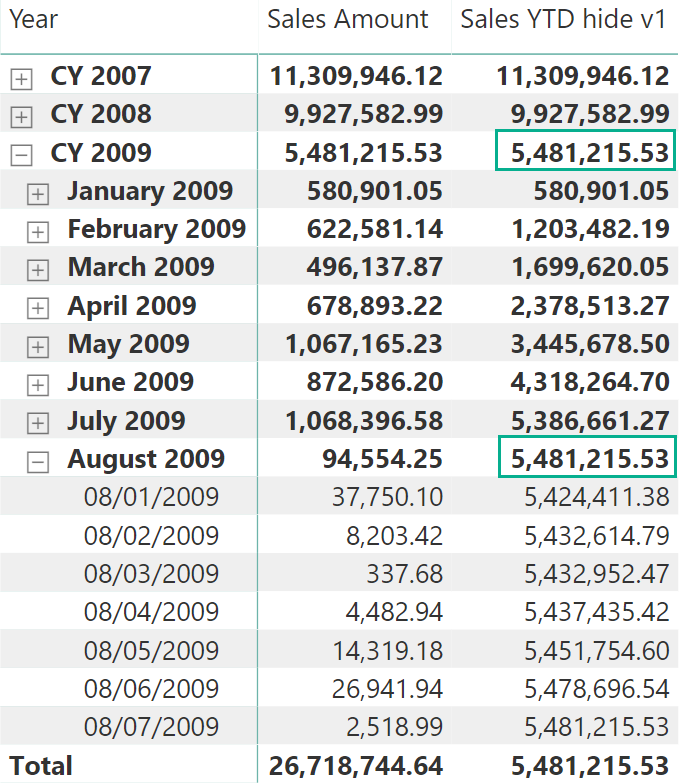The year-over-year (YOY) calculation is also implemented by correctly defining the previous year (PY) measure, referencing it in a new version of YOY %. The result in the following screenshot correctly computes the amount for August 2009 and CY 2009.

```Sales PY hide v1 =
CALCULATE (
[Sales Amount],
CALCULATETABLE (
SAMEPERIODLASTYEAR ( 'Date'[Date] ),
'Date'[DatesWithSales] = TRUE
)
)

Sales YOY % hide v1 =
VAR CurrentSales = [Sales Amount]
VAR PreviousSales = [Sales PY hide v1]
VAR DeltaSales = CurrentSales - PreviousSales
VAR Result =
IF (
NOT ISBLANK ( CurrentSales ),
DIVIDE ( DeltaSales, PreviousSales )
)
RETURN Result
```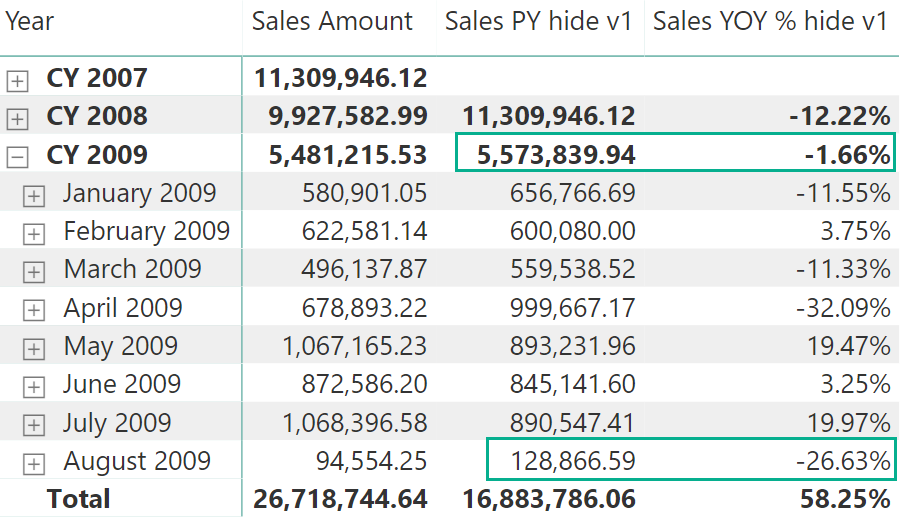The same approach can be applied by comparing the year-to-date of the current period (YTD) with the year-to-date of the previous year (PYTD), displaying a percentage difference between the current year-to-date and the year-to-date of the previous year (YOYTD %).

```Sales PYTD v1 =
CALCULATE (
[Sales YTD hide v1],
CALCULATETABLE (
SAMEPERIODLASTYEAR ( 'Date'[Date] ),
'Date'[DatesWithSales]
)
)

Sales YOYTD % v1 =
VAR CurrentSales = [Sales YTD hide v1]
VAR PreviousSales = [Sales PYTD v1]
VAR DeltaSales = CurrentSales - PreviousSales
VAR Result =
IF (
NOT ISBLANK ( CurrentSales ),
DIVIDE ( DeltaSales, PreviousSales )
)
RETURN Result
```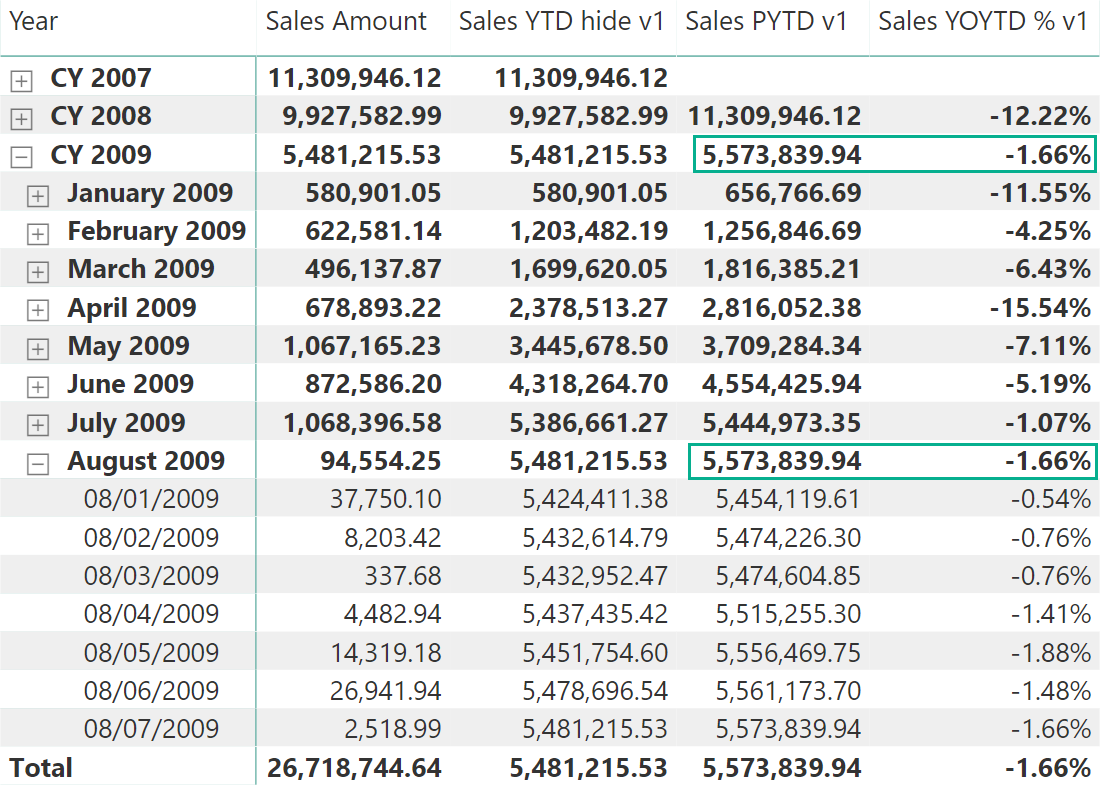The solution of using a calculated column can be considered a best practice, because it provides good performance and it requires the minimum amount of code in DAX, following a simple and effective pattern calling time intelligence functions.

This solution cannot be used when the last date to consider is dynamic (for example because it is different for different measures). However, if it is possible to create one calculated column for each related fact table that may have a different range of dates to consider, doing so is still a good idea. Every measure will use the flag in the Date table corresponding to the proper fact table. For example, a DatesWithSales flag can be used in Sales measures, whereas a DatesWithPurchases flag can be used in Purchases measures.

If the data model cannot be modified, then a different approach based entirely on measures is required. This is the topic of the next section.

## Filtering dates using measures alone

There is a different approach in filtering future dates that obtains the same results by just using measures, without relying on a calculated column defined in the Date table. Whenever possible, leveraging the calculated column is a better choice, providing better performance and simpler DAX code. However, if you connect Power BI to an external model using a live connection, you can only create local report measures and you are unable to modify the data model. In such cases, you need to pay more attention to the DAX code to write, which will be slightly slower and more complex.

You will see two techniques based on DAX measures. The technique with the suffix v2 might work in some scenarios but not in others. That technique is shown for educational purposes, because it could be considered more intuitive and easier to write, but it also hides several pitfalls. The technique with the suffix v3 is more reliable and it is the suggested technique in case the measures with the suffix v1 cannot be used.

### Hiding dates using IF

By comparing the dates in the filter context with the last day available in the Sales table, it is possible to hide the result of a calculation in the report. This may be enough for a calculation like year-to-date (YTD):

```Sales YTD hide v2 =
VAR LastDayAvailable =
CALCULATE (
MAX ( Sales[Order Date] ),
ALL ( Sales )
)
VAR FirstDayInSelection =
MIN ( 'Date'[Date] )
VAR ShowData =
(FirstDayInSelection <= LastDayAvailable)
VAR Result =
IF (
ShowData,
CALCULATE (
[Sales Amount],
DATESYTD ( 'Date'[Date] )
)
)
RETURN Result
```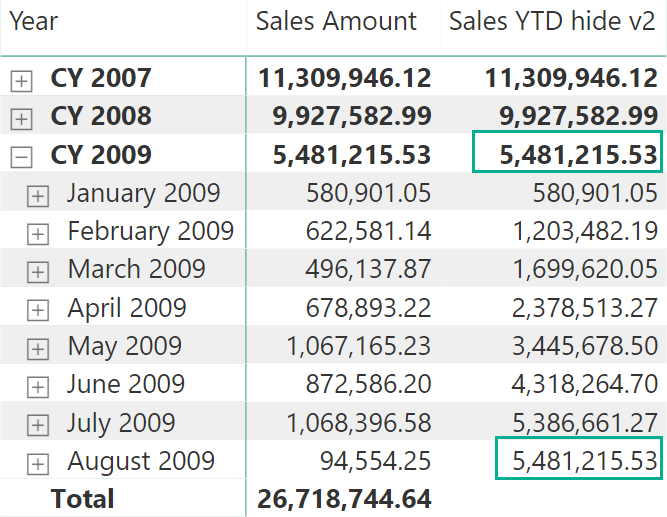However, this approach does not work well for the year-over-year comparison (YOY %), as shown in the following example:

```Sales PY hide v2 =
VAR LastDayAvailable =
CALCULATE (
MAX ( Sales[Order Date] ),
ALL ( Sales )
)
VAR FirstDayInSelection =
MIN ( 'Date'[Date] )
VAR ShowData =
(FirstDayInSelection <= LastDayAvailable)
VAR Result =
IF (
ShowData,
CALCULATE (
[Sales Amount],
SAMEPERIODLASTYEAR ( 'Date'[Date] )
)
)
RETURN Result

Sales YOY % hide v2 =
VAR CurrentSales = [Sales Amount]
VAR PreviousSales = [Sales PY hide v2]
VAR DeltaSales = CurrentSales - PreviousSales
VAR Result =
IF (
NOT ISBLANK ( CurrentSales ),
DIVIDE ( DeltaSales, PreviousSales )
)
RETURN Result
```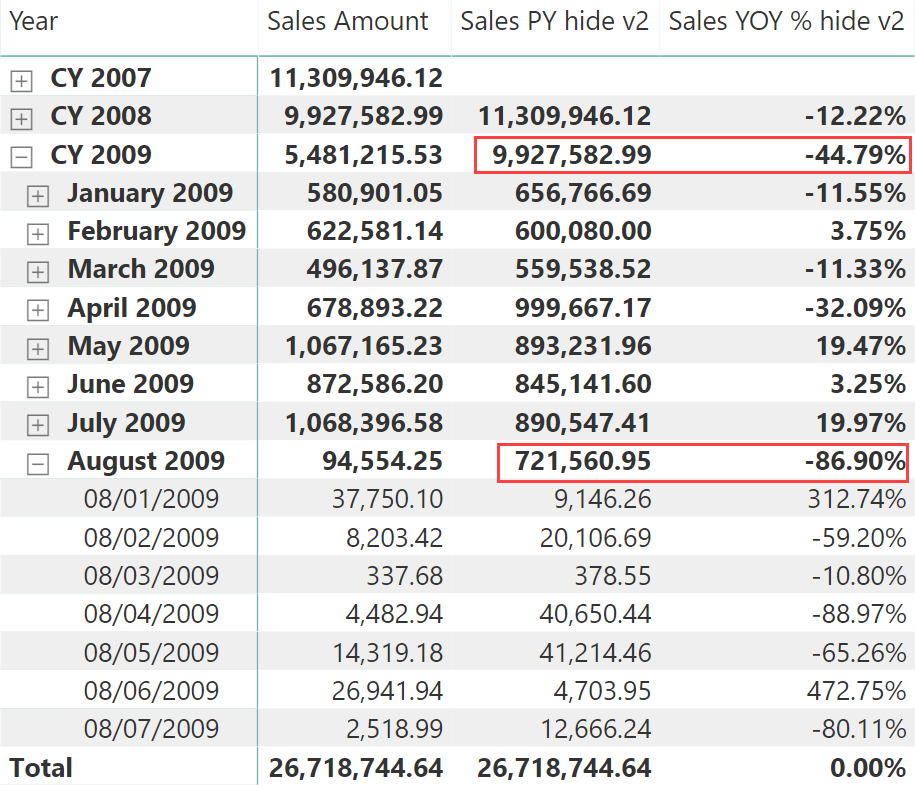The only effect of this calculation is to hide the dates greater than August 7, 2009 in the report. However, the previous year (PY) values computed for August 2009 and CY 2009 are wrong, because they consider the entire period (one month and one year, respectively) instead of only considering the days until August 7 in 2008.

Similar issues exist when comparing the year-to-date of the previous year (PYTD). Calculating the Sales YTD measure after applying the time intelligence function would miss the logic implemented in the IF function of such internal measure, because Sales YTD would be executed in a filter context of the year 2008, where all the dates are present:

```Sales PYTD v2 =
CALCULATE (
[Sales YTD hide v2],
SAMEPERIODLASTYEAR ( 'Date'[Date] )
)

Sales YOYTD % v2 =
VAR CurrentSales = [Sales YTD hide v2]
VAR PreviousSales = [Sales PYTD v2]
VAR DeltaSales = CurrentSales - PreviousSales
VAR Result =
IF (
NOT ISBLANK ( CurrentSales ),
DIVIDE ( DeltaSales, PreviousSales )
)
RETURN Result
```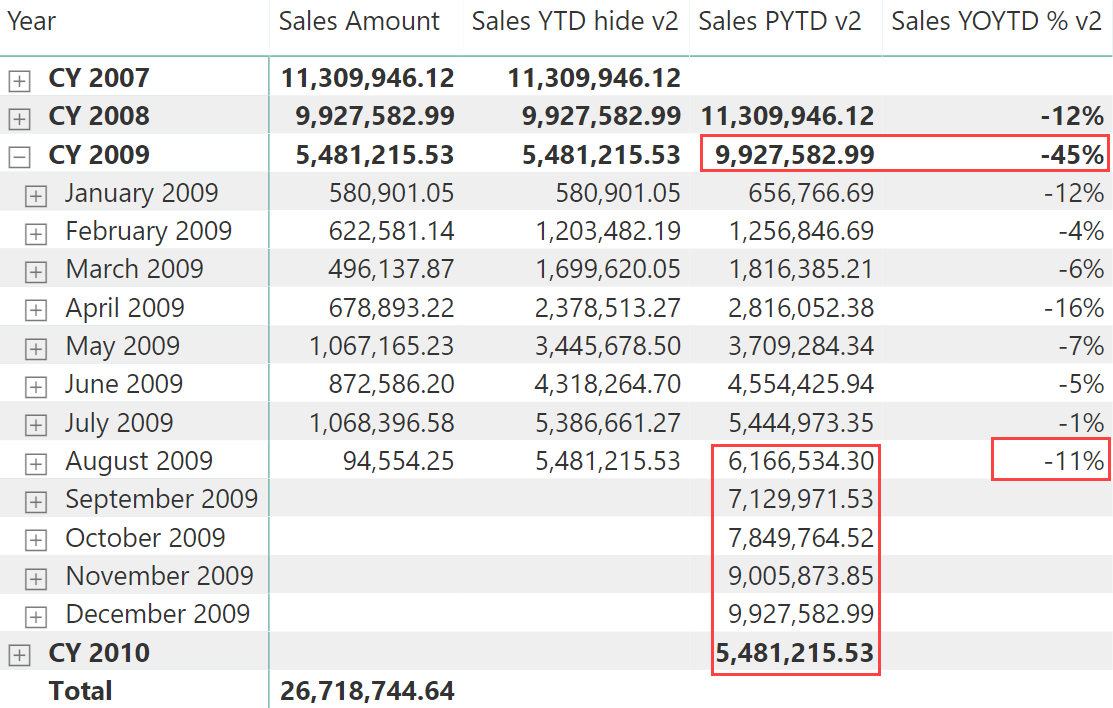The YOYTD% measure is wrong for CY 2009 and for August 2009, because it considers a value for PYTD that includes dates of 2008 that have no corresponding sales in 2009. Trying to capture the filter context managing it with IF functions creates longer DAX code that is not easy to encapsulate in measures called by other measures.

### Hiding dates by intercepting the filter context

A different approach is possible by modifying the dates in the filter context before passing them to the time intelligence functions. Usually, a time intelligence function just receives a date column reference:

```SAMEPERIODLASTYEAR ( 'Date'[Date] )
```

However, the syntax above is just syntax sugar for this internal evaluation – a time intelligence function always receives a table with dates:

```SAMEPERIODLASTYEAR ( CALCULATETABLE ( VALUES ( 'Date'[Date] ) ) )
```

The CALCULATETABLE function can be ignored when the time intelligence function is not called in a row context. Therefore, every call to a time intelligence function using a column reference simply copies the dates visible in the filter context in a table passed as an argument. When we provide a table as an argument, we directly control the dates passed to the time intelligence function.

We can filter the dates in the filter context by removing the dates that are in the future before calling the time intelligence function. For example, this is the implementation of the year-to-date (YTD) using this technique:

```Sales YTD hide v3 =
VAR LastDayAvailable =
CALCULATE (
MAX ( Sales[Order Date] ),
ALL ( Sales )
)
VAR CurrentDates =
FILTER (
VALUES ( 'Date'[Date] ),
'Date'[Date] <= LastDayAvailable
)
VAR Result =
CALCULATE (
[Sales Amount],
DATESYTD ( CurrentDates )
)
RETURN Result
```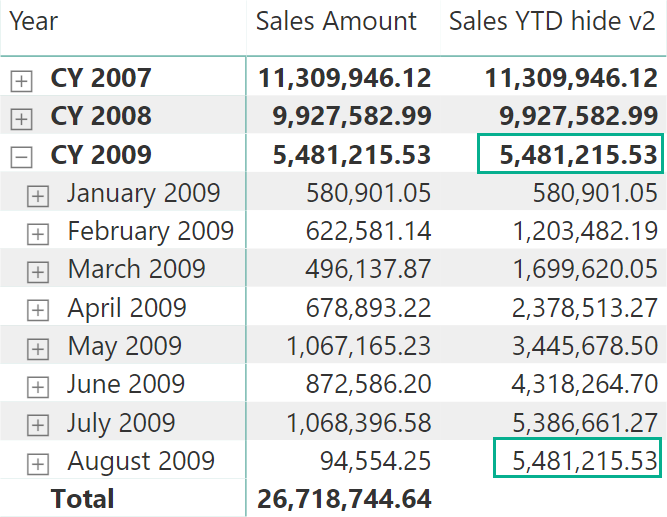The same approach works well for the year-over-year (YOY) calculation:

```Sales PY hide v3 =
VAR LastDayAvailable =
CALCULATE (
MAX ( Sales[Order Date] ),
ALL ( Sales )
)
VAR CurrentDates =
FILTER (
VALUES ( 'Date'[Date] ),
'Date'[Date] <= LastDayAvailable
)
VAR Result =
CALCULATE (
[Sales Amount],
SAMEPERIODLASTYEAR ( CurrentDates )
)
RETURN Result

Sales YOY % hide v3 =
VAR CurrentSales = [Sales Amount]
VAR PreviousSales = [Sales PY hide v3]
VAR DeltaSales = CurrentSales - PreviousSales
VAR Result =
IF (
NOT ISBLANK ( CurrentSales ),
DIVIDE ( DeltaSales, PreviousSales )
)
RETURN Result
```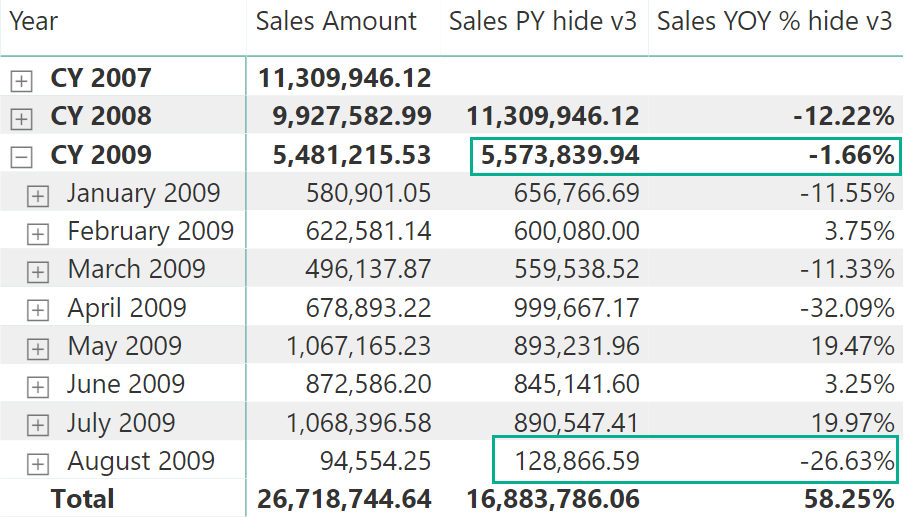Finally, the same technique can be applied when comparing with the year-to-date of the previous year:

```Sales PYTD v3 =
VAR LastDayAvailable =
CALCULATE (
MAX ( Sales[Order Date] ),
ALL ( Sales )
)
VAR CurrentDates =
FILTER (
VALUES ( 'Date'[Date] ),
'Date'[Date] <= LastDayAvailable
)
VAR Result =
CALCULATE (
[Sales YTD hide v3],
SAMEPERIODLASTYEAR ( CurrentDates )
)
RETURN Result

Sales YOYTD % v3 =
VAR CurrentSales = [Sales YTD hide v3]
VAR PreviousSales = [Sales PYTD v3]
VAR DeltaSales = CurrentSales - PreviousSales
VAR Result =
IF (
NOT ISBLANK ( CurrentSales ),
DIVIDE ( DeltaSales, PreviousSales )
)
RETURN Result
```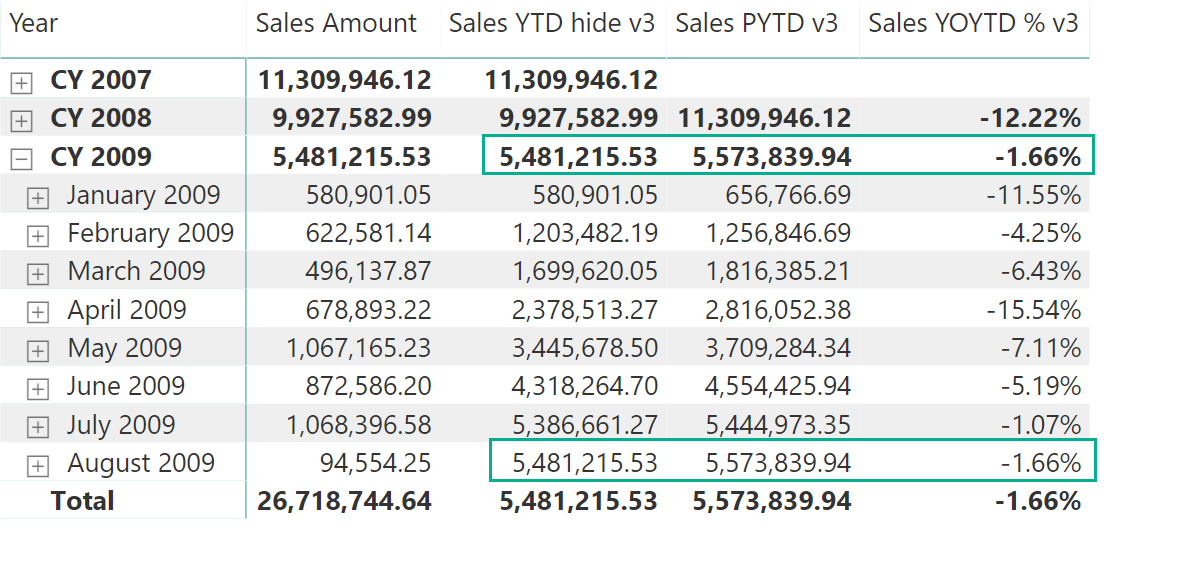## Conclusions

Whenever possible, use one or more calculated columns in the Date table to filter out future dates calling time intelligence functions. If this is not possible because you cannot modify the data model, implement the same logic in DAX measures, providing a filtered table of dates to the time intelligence functions. These solutions are better than IF conditions that could increase the complexity of both DAX code and query plan, with possible side effects on performance.

SAMEPERIODLASTYEARContext transition

Returns a set of dates in the current selection from the previous year.

`SAMEPERIODLASTYEAR ( <Dates> )`

IFChecks whether a condition is met, and returns one value if TRUE, and another value if FALSE.

`IF ( <LogicalTest>, <ResultIfTrue> [, <ResultIfFalse>] )`

CALCULATETABLEContext transition

Evaluates a table expression in a context modified by filters.

`CALCULATETABLE ( <Table> [, <Filter> [, <Filter> [, … ] ] ] )`